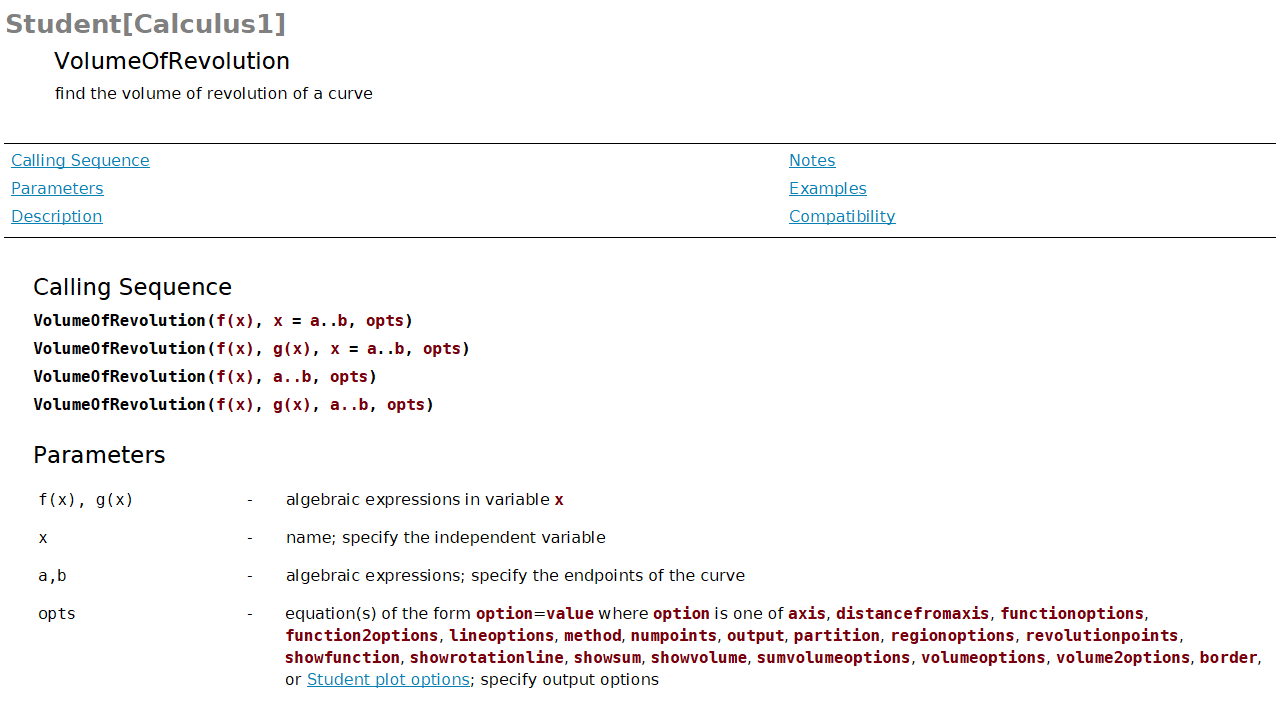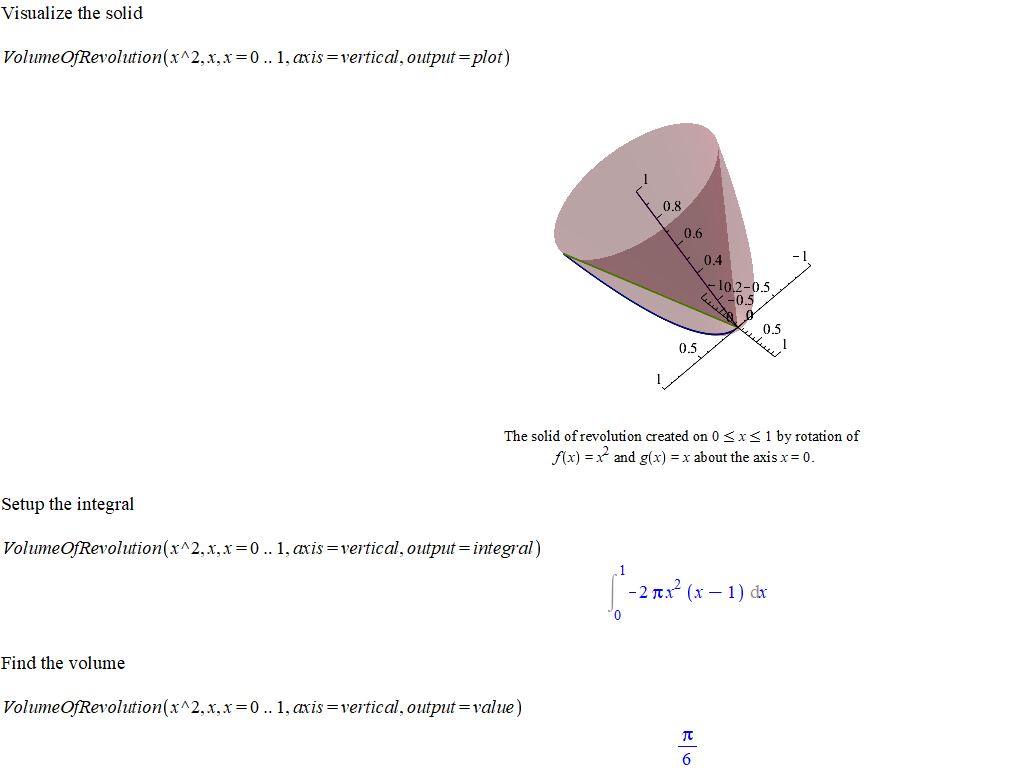# Topic 7 Applications of Integrals II

## 7.1 Volume of Revolution

In terms of definite integrals, the volume of a solid obtained by rotating a region about the $$x$$-axis can be calculated by $\int_a^b \pi (r_1(x)^2 - r_2(x)^2) \mathrm{d} x \qquad \text{disk/washer method},$ or $\int_a^b 2\pi r(y) h(y) \mathrm{d} y \qquad \text{shell method method},$

where $$r_1(x)$$, $$r_2(x)$$ and $$r(x)$$ represents the radius and $$h(x)$$ represents the height of a cylindrical shell.

In practice, it’s better to recognize the shape of a cross section, find the volume of a slice of the solid and then set up the integral.

In the following, you will see some tools/commands from Maple which are very helpful to calculate the volume of a solid.

In Maple, the following command, supported by the package Student[Calculus1], can be used to get the graph, the integral and the volume of the solid obtained by rotation the region bounded by $$f(x)$$, $$g(x)$$, $$x=a$$ and $$x=b$$.

VolumeOfRevolution(f(x), g(x), x = a..b, opts)

To learn what options does the command VolumeOfRevolution have, you may type

?VolumeOfRevolution

in the Math mode and hit enter. You will see the help page.Maple help page for VolumeOfRevolution command

Example 7.1 Show the solid obtained by rotating the region bounded by $$y=x^2$$ and $$y=x$$ about $$y$$-axis. Set up an integral for the volume. Find the volume.

Solution.

with(Student[Calculus1])

#Show the solid

VolumeOfRevolution(x^2, x, x = 0 .. 1, axis = vertical, output = plot)

#Set up an integral

VolumeOfRevolution(x^2, x, x = 0 .. 1, axis = vertical, output = integral)

#Find the volume

VolumeOfRevolution(x^2, x, x = 0 .. 1, axis = vertical, output = value)

The outputs in Maple can be seen in the following pictureVolume of Revolution Example

Remark. 1. If you change the function to VolumeOfRevolutionTutor, you will see an interactive popup windows which does exactly the same thing.

1. If the rotation axis is not an axis of the coordinate system, you need add the option distancefromaxis = numeric into the function. For example, if in the above example, the rotation is about $$y=-2$$, then the Maple command should be the following

 VolumeOfRevolution(x^2, x, x = 0 .. 1, axis = vertical, distancefromaxis = -2, output = integral)
Exercise 7.1 Find the volume of the solid obtained by rotating the region bounded by $$y=x^3$$, $$x=0$$, $$y=1$$ about $$y$$-axis
Exercise 7.2 Find the volume of the solid obtained by rotating the region bounded by $$y=x^3$$, $$y=0$$, $$x=1$$ about (a) $$y=0$$, and (b) $$x=2$$.